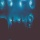Home
IT Knowledge
Inspiration
Languages
EN

# Python - replace all occurrences of string

0 points
Created by:Lennox-A
395

In this article, we would like to show you how to replace all occurrences of string in Python.

Quick solution:

``````text = "ABC ABC ABC"
replacement = "X"
result = text.replace("C", replacement)

print(result)  # ABX ABX ABX``````

## 1. Practical example using String `replace()` method

In this example, we use String `replace()` method to replace all occurrences of the "`C`" letter in the `text` string.

``````text = "ABC ABC ABC"
replacement = "X"
result = text.replace("C", replacement)

print(result)  # ABX ABX ABX``````

Output:

``ABX ABX ABX``

Note:

Optionally you can add the third argument that specifies how many occurrences of the old value you want to replace. By default the `replace()` method replaces all occurrences.

## 2. Practical example using String `replace()` method with optional argument

In this example, we use String `replace()` method with the third (`count`) argument to replace `2` occurrences of the "`C`" letter in the `text` string.

``````text = "ABC ABC ABC"
replacement = "X"
count = 2
result = text.replace("C", replacement, count)

print(result)  # ABX ABX ABC``````

Output:

``ABX ABX ABC``